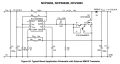# Calculating the peak current of my boost converter

#### Electronic_Maniac

Joined Oct 26, 2017
252

I am stuck while calculating the peak current in the boost converter through the inductor.

Can you please check if I am right?

Ipeak=(Max Voltage/ R2) = 18/1000=0.018. So, my peak current through the inductor is just 18mA? Am I doing it correct. If this is correct, how will I account for the Vce drop inside the pin1 and pin2 of the IC. The darlington transistor inside the IC is just like a normal transistor. I have added two transistor outside the IC instead of using the darlington switch in the IC for thermal purposes.

Can you please help me with the calculation of Vswce and Ipeak. The calculation of Vswce is not mentioned in the datasheet. So, please help. And the calculation of Peak current is mentioned in the datasheet. But is my approach of calculating the peak current through the inductor right?Last edited by a moderator:

#### Jony130

Joined Feb 17, 2009
5,145
For the circuit given in figure 15 the Ipk = ((R2 + R3)0.2V - R2 Vin)/(R1*R3).

As for Vswce = 1V....1.3V max

•Electronic_Maniac